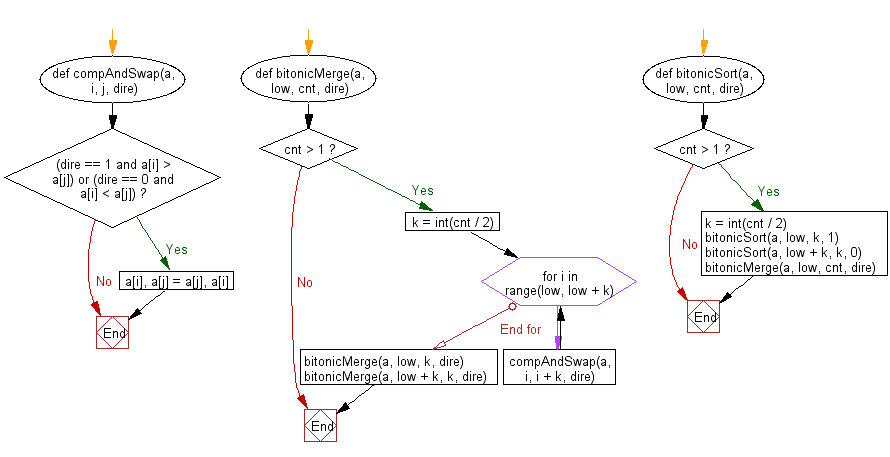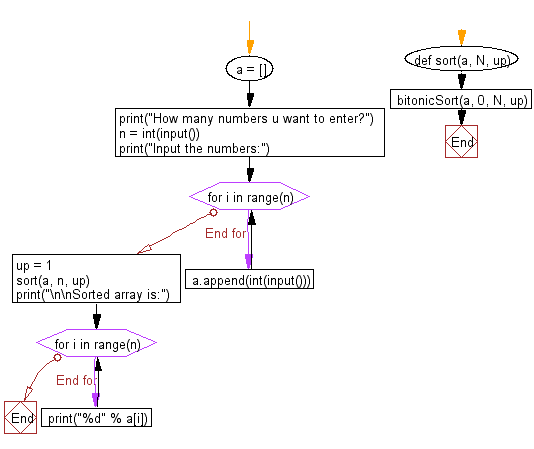﻿ Python: Create a program for Bitonic Sort - w3resource# Python: Create a program for Bitonic Sort

## Python Search and Sorting : Exercise-11 with Solution

Write a Python code to create a program for Bitonic Sort.
Bitonic Sort: According to rutgers.edu - Bitonic sort is a comparison-based sorting algorithm that can be run in parallel. It focuses on converting a random sequence of numbers into a bitonic sequence, one that monotonically increases, then decreases. Rotations of a bitonic sequence are also bitonic.
More specifically, bitonic sort can be modelled as a type of sorting network. The initial unsorted sequence enters through input pipes, where a series of comparators switch two entries to be in either increasing or decreasing order.
The algorithm, created by Ken Batcher in 1968, consists of two parts. First, the unsorted sequence is built into a bitonic sequence; then, the series is split multiple times into smaller sequences until the input is in sorted order.

Sample Solution:

Python Code:

``````#License:  https://bit.ly/2InTS3W
# Python program for Bitonic Sort. Note that this program
# works only when size of input is a power of 2.
# The parameter dir indicates the sorting direction, ASCENDING
# or DESCENDING; if (a[i] > a[j]) agrees with the direction,
# then a[i] and a[j] are interchanged.*/
def compAndSwap(a, i, j, dire):
if (dire == 1 and a[i] > a[j]) or (dire == 0 and a[i] < a[j]):
a[i], a[j] = a[j], a[i]
# It recursively sorts a bitonic sequence in ascending order,
# if dir = 1, and in descending order otherwise (means dir=0).
# The sequence to be sorted starts at index position low,
# the parameter cnt is the number of elements to be sorted.
def bitonicMerge(a, low, cnt, dire):
if cnt > 1:
k = int(cnt / 2)
for i in range(low, low + k):
compAndSwap(a, i, i + k, dire)
bitonicMerge(a, low, k, dire)
bitonicMerge(a, low + k, k, dire)

# This funcion first produces a bitonic sequence by recursively

# sorting its two halves in opposite sorting orders, and then
# calls bitonicMerge to make them in the same order
def bitonicSort(a, low, cnt, dire):
if cnt > 1:
k = int(cnt / 2)
bitonicSort(a, low, k, 1)
bitonicSort(a, low + k, k, 0)
bitonicMerge(a, low, cnt, dire)

# Caller of bitonicSort for sorting the entire array of length N

# in ASCENDING order
def sort(a, N, up):
bitonicSort(a, 0, N, up)

# Driver code to test above
a = []
print("How many numbers u want to enter?");
n = int(input())
print("Input the numbers:");
for i in range(n):
a.append(int(input()))
up = 1

sort(a, n, up)
print("\n\nSorted array is:")
for i in range(n):
print("%d" % a[i])
```
```

Sample Output:

```How many numbers u want to enter?
5
Input the numbers:
25
13
76
59
37

Sorted array is:
13
25
59
76
37
```

Flowchart:Python Code Editor:

What is the difficulty level of this exercise?

Test your Programming skills with w3resource's quiz.

﻿

## Python: Tips of the Day

The Zip() Function:

```>>> students = ('John', 'Mary', 'Mike')
>>> ages = (15, 17, 16)
>>> scores = (90, 88, 82, 17, 14)
>>> for student, age, score in zip(students, ages, scores):
...     print(f'{student}, age: {age}, score: {score}')
...
John, age: 15, score: 90
Mary, age: 17, score: 88
Mike, age: 16, score: 82
>>> zipped = zip(students, ages, scores)
>>> a, b, c = zip(*zipped)
>>> print(b)
(15, 17, 16)
```